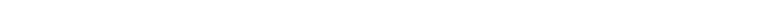Textbook Notes (280,000)
CA (170,000)
U of G (10,000)
SOAN (400)
SOAN 2120 (100)
Chapter 8

# SOAN 2120 Chapter Notes - Chapter 8: Precoding, Scantron Corporation, Codebook

Department
Sociology and Anthropology
Course Code
SOAN 2120
Professor
William Walters
Chapter
8

This preview shows pages 1-2. to view the full 7 pages of the document.Chapter Eight
Coding Data
Coding: Systematically reorganizing raw numerical data into a format that is
easy to analyze using computers.
Codebook: A document (one or more pages) describing the coding
procedure and the location of data for variables in a format that a computer
can use.
Pre-coding: Placing code categories on the questionnaire. If this is not done,
the first step is to create a codebook.
Entering Data
There are four ways to get raw quantitative data into a computer:
Code Sheet: Gather the info, and then transfer it from the original
source onto a code sheet. Then type line by line into computer.
Direct-Entry Method, Including CATI: Type the information or get
the respondent to type the information in the computer. The
computer must be preprogrammed to accept answers.
Optical Scan: Enter the information onto optical scan sheets (like a
Scantron), which will then transfer the info into the computer.
Bar Code: Convert the info into different widths of bars associated
with specific numerical values; use a bar code reader to transfer the
info into the computer.
Cleaning Data
After careful coding, the researcher verifies the accuracy of coding, or
“cleans” the data.
Researchers verify the codes in two ways:
Possible code cleaning (or wild code checking): involves checking
the categories of all variables for impossible codes
Contingency Cleaning (or Consistency Checking): involves
cross-classifying two variables and looking for logically impossible
combinations
Results with One Variable
Frequency Distributions
Descriptive Statistics: Numerical data
Can be categorized by number of variables involved
(univariate, bivariate, or multivariate)
Univariate: describes one variable. To describe this, we use
frequency distribution (nominal-, ordinal-, interval- or
ratio-level data and takes many forms)
Histograms, bar charts and pie charts are great representation
of these.

Only pages 1-2 are available for preview. Some parts have been intentionally blurred.For interval or ration level data, info is often formed into
categories and is plotted in a frequency polygon. (Frequency
along y-axis, values of variable/score around x-axis)
Measures of Central Tendency
Mode: Most common/frequent number
Median: Middle number once arranged numerically or if there’s two middle
numbers, both numbers added then divided by 2.
Mean: All numbers added then divided by the number of numbers.
Measures of Variation
Definition: A one-number summary of a distribution (its center).
Distributions also have spread, dispersion or variability
Two distributions can have identical measures of central tendency but
Variation
Zero Variation: means that every person in the population has the
median and mean value.
Measured in three ways
Range: Largest and smallest scores. E.g. a bar has ages 19-26 in
it. The range is then 7.
Percentile: Tell the score at a specific place within the
distribution. The median is the 50th percentile.
Standard Deviation: Requires the interval or ratio level of
management. Gives an average distance between all scores
and the mean.
Normal distribution looks like a hill
How to calculate standard deviation (recap of in-class notes)
1. Compute the mean
2. Subtract the mean from each score
3. Square the resulting difference for each score
4. Total up the squared differences to get the sum of squares.
5. Divide the sum of squares by the number of cases minus 1 to
get the variance
6. Take the square root of the variance, which is the standard
deviation
Calculating Z-Scores
1. Z-Score = (Score-Mean)/Standard Deviation
---------------------------------------------
Standard Deviation
Results with Two Variables
Bivariate Statistics: Let a researcher consider two variables together and
describe the relationship between variables.